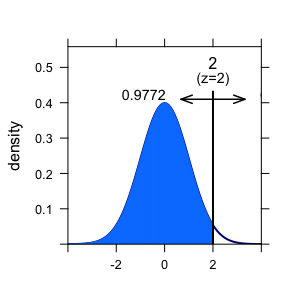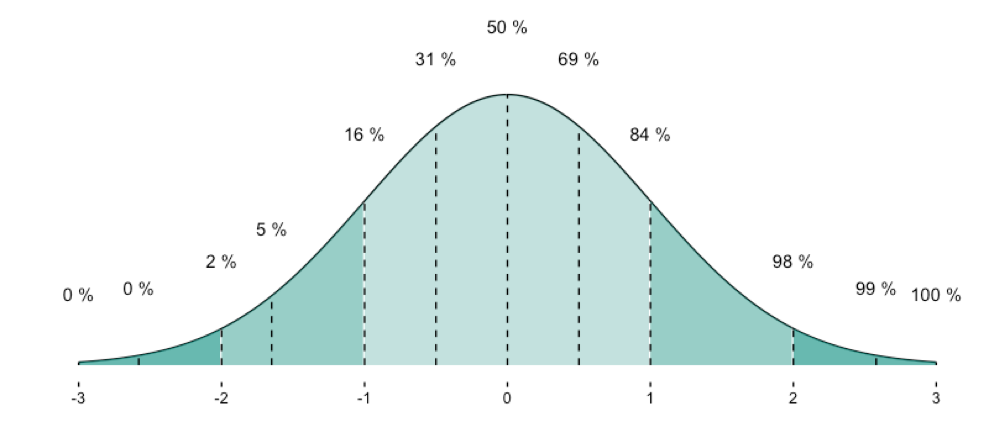# Why have z-transformed values a mean of zero and a sd of 1?

## July 02, 2016

Reading time ~4 minutes

z-transformation is an ubiquitous operation in data analysis. It is often quite practical.

Example: Assume Dr Zack scored 42 points on a test (say, IQ). Average score is 40 in the relevant population, and SD is 1, let’s say. So Zack’s score is 2 points above average. 2 points equals to SDs in this example. We can thus safely infer that Zack is about 2 SDs above average (leaving measurement precision and other issues at side).

If the variable (IQ) is normally distributed, than we are allowed to look up the percentiles of this z-value in a table. Or ask the computer (in R):

pnorm(2)

##  0.9772499


Here, R tells us that approx. 98% of all individuals have an IQ that is smaller than that of Dr. Zack. Lucky guy! Compare the figure.Here an overview on some frequently used quantiles of the normal distribution:Now, next step. We have a bunch of guys, and test each of them. Say, 10 guys. Now we can calculate mean and SD of this distribution. To see the differences more clearly, we can z-transform the values.

IQ <- rnorm(10, mean = 40, sd = 1)
IQ

##   40.91472 38.53260 40.30042 40.87957 40.99679 39.86815 40.14272
##   39.26335 40.19174 39.85837

IQ_z <- scale(IQ)
IQ_z

##              [,1]
##  [1,]  1.05897590
##  [2,] -2.01783361
##  [3,]  0.26552871
##  [4,]  1.01356631
##  [5,]  1.16497561
##  [6,] -0.29280567
##  [7,]  0.06183789
##  [8,] -1.07397171
##  [9,]  0.12515803
## [10,] -0.30543146
## attr(,"scaled:center")
##  40.09484
## attr(,"scaled:scale")
##  0.7742196


Ok. Let’s compute mean and sd of the z-scaled values:

mean(IQ_z)

##  -4.605338e-15

sd(IQ_z)

##  1


So it worked apparently.

But why is it that mean and sd will end up nicely with 0 (mean) and 1 (sd)?

To see this, consider the following. A z-transformation is in step 1 nothing more than subtracting the mean of some value. If I subtract the mean (42 here) from each value, and compute the mean then, I will not be surprised that the mean is now 42 points lower than it was. But 42-42=0. So the new mean (after z-transformation) will be 0.

Ok, but what about sd? Why must it equal 1? A similar reasoning applies. If I divide each value by the SD of the distribution (here 1), the new SD will be exactly by this factor lower. So whatever it used to be before z-transformtion, it will be 1 afterwards, as x / x = 1.

### New bar stacking with ggplot 2.2.0

Recently, ggplot2 2.2.0 was released. Among other news, stacking bar plot was improved. Here is a short demonstration.Load libraries`...… Continue reading

#### Crashkurs zur Erstellung von Barplots für Umfrage-Daten

Published on November 13, 2016

#### Some thoughts (and simulation) on overfitting

Published on November 12, 2016